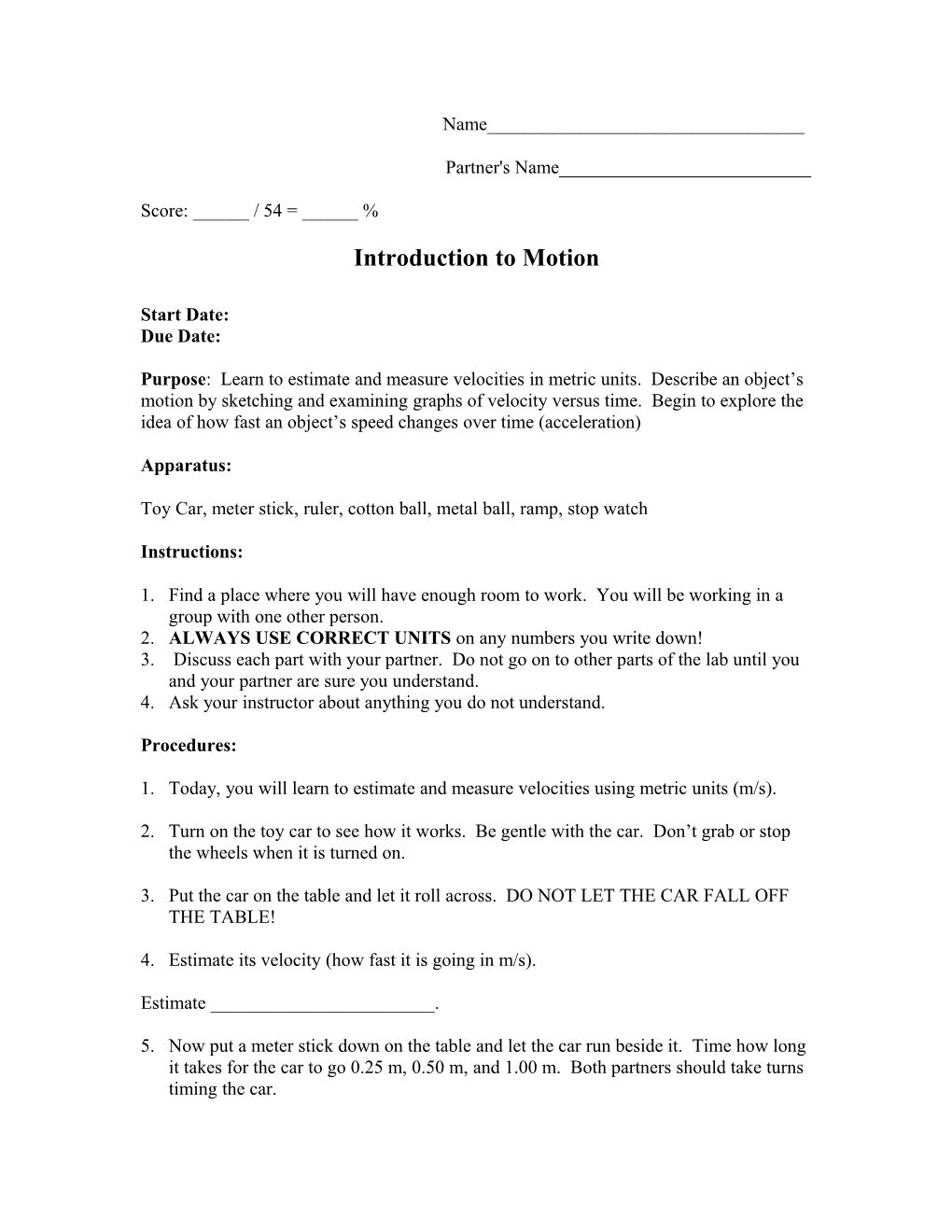# Introduction to MotionName______

Partner's Name______

Score: ______/ 54 = ______%

## Introduction to Motion

Start Date:
Due Date:

Purpose: Learn to estimate and measure velocities in metric units. Describe an object’s motion by sketching and examining graphs of velocity versus time. Begin to explore the idea of how fast an object’s speed changes over time (acceleration)

Apparatus:

Toy Car, meter stick, ruler, cotton ball, metal ball, ramp, stop watch

Instructions:

1. Find a place where you will have enough room to work. You will be working in a group with one other person.
2. ALWAYS USE CORRECT UNITS on any numbers you write down!
3. Discuss each part with your partner. Do not go on to other parts of the lab until you and your partner are sure you understand.

Procedures:

1. Today, you will learn to estimate and measure velocities using metric units (m/s).
1. Turn on the toy car to see how it works. Be gentle with the car. Don’t grab or stop the wheels when it is turned on.
1. Put the car on the table and let it roll across. DO NOT LET THE CAR FALL OFF THE TABLE!
1. Estimate its velocity (how fast it is going in m/s).

Estimate ______.

1. Now put a meter stick down on the table and let the car run beside it. Time how long it takes for the car to go 0.25 m, 0.50 m, and 1.00 m. Both partners should take turns timing the car.
1. Fill the data you collect into the data table below.
1. Find the velocity of all three trips.

Distance / 0.25 m / 0.50 m / 1.00 m
Time
Velocity

Answer question 1: Are the velocities for the three trips about the same? If not, why aren’t they the same?

1. Sketch a graph of distance versus time for the car. This should be done on a separate sheet of paper. Use up as much of the paper as you can while making your graph. Use a ruler to draw straight lines and make sure you divide up each axis evenly according to the range of data.

Answer question 4: Using the graph, predict how far the car will go in 2 seconds, 15 seconds, and 45 seconds. You will have to use interpolation and extrapolation to do this.

1. Now, measure how far it goes in 15 seconds.

Measurement ______

Answer question 7: Figure out how to calculate the distance the car will go for any given period of time? Please tell me what equation you would use to do this. (Just do your best with this one!)

1. Now you will learn how to describe an object’s motion by sketching graphs of distance versus time. Observe the motions of the objects described below using the materials on your desk. Describe the motion in words. Then, sketch a rough graph of distance versus time for each. Again, just do a rough sketch and don’t worry about plotting data points. Compare your graphs with your partner’s.
1. Watch the toy car drive across the table.
1. Drop the metal ball from as high as you can reach.
1. Drop the cotton ball from as high as you can reach.
1. Now let’s think about how fast an object’s speed changes (acceleration). Since there is only one ramp available, the teacher will do this as a demonstration for the class. Look at the ramp at the front of the room. When the ball is allowed to roll down the ramp, its speed is not constant.
1. Its initial speed (Vi) is zero. It speeds up and reaches a final speed when it hits the table. The final speed (Vf) of the ball can be measured by measuring the nearly constant speed it has when it hits then rolls on the table.
1. First, the ball will be rolled down the table. Before any measurements are taken, estimate the final velocity using units of m/s.

Estimate ______

1. Now, together the class will measure the velocity of the ball, on the table, over a distance of about 2 meters.

Measurement ______

1. The speed that it gained (∆V) be going down the ramp is calculated by subtracting the initial speed from the final speed.

∆V ______

1. Now, if we divide the speed it gained (∆V) by the time it was speeding up, we have the acceleration!

Answer question 8: So, what was the acceleration (a) of the ball going down the ramp?

Acceleration ______

Answer question 9: What are the units for acceleration?

Calculations:

Show all calculations on a separate sheet of paper.

Conclusions: Don’t forget to make conclusions on your lab report!